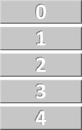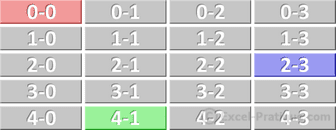# VBA Course: Variables (continued)

## Arrays

While variables only allow us to store one value each, arrays make it possible to store many values and they work almost exactly the same way.

Here are some examples of declarations:

``````'Sample variable declaration
Dim var1 As String

'Sample 1 dimensional array declaration
Dim array1(4) As String

'Sample 2 dimensional array declaration
Dim array2(4, 3) As String

'Sample 3 dimensional array declaration
Dim array3(4, 3, 2) As String``````

The 1 dimensional array:

``````'Sample 1 dimensional array declaration
Dim array1(4) As String``````

There is only one number in parentheses in this declaration, which means that it is a one dimensional array. This number sets the size of the array. array1(4) is an array in which the cells are numbered from 0 to 4, which means that it is an array with 5 cells:

``````'Assigning values to the 5 cells
array1(0) = "Value of cell 0"
array1(1) = "Value of cell 1"
array1(2) = "Value of cell 2"
array1(3) = "Value of cell 3"
array1(4) = "Value of cell 4"``````Important: the first cell in an array is numbered 0.

Another example, a two dimensional array:

``````'Sample declaration of a 2 dimensional array
Dim array2(4, 3) As String````````````'Assigning values to three colored cells
array2(0, 0) = "Red cell value"
array2(4, 1) = "Green cell value"
array2(2, 3) = "Blue cell value"``````

## Constants

Like variables, constants can be used to store values, but the difference is that they can't be modified (thus their name).

For example, we could add a constant to avoid having to repeat a number like 6.87236476641:

``````Sub const_example()
Cells(1, 1) = Cells(1, 2) * 6.87236476641
Cells(2, 1) = Cells(2, 2) * 6.87236476641
Cells(3, 1) = Cells(3, 2) * 6.87236476641
Cells(4, 1) = Cells(4, 2) * 6.87236476641
Cells(5, 1) = Cells(5, 2) * 6.87236476641
End Sub``````

This makes the code much easier to read (important parts in particular) and makes it much easier to change the value of the constant, should you need to:

``````Sub const_example()
'Declaration of a constant + assignment of value
Const ANNUAL_RATE As Double = 6.87236476641

Cells(1, 1) = Cells(1, 2) * ANNUAL_RATE
Cells(2, 1) = Cells(2, 2) * ANNUAL_RATE
Cells(3, 1) = Cells(3, 2) * ANNUAL_RATE
Cells(4, 1) = Cells(4, 2) * ANNUAL_RATE
Cells(5, 1) = Cells(5, 2) * ANNUAL_RATE
End Sub``````

## The scope of variables

If a variable is declared at the beginning of a procedure (Sub), it can only be used within this same procedure. The value of the variable will not be maintained after the execution of the procedure.

``````Sub procedure1()
Dim var1 As Integer
' => Use of a variable only within a procedure
End Sub

Sub procedure2()
' => var1 cannot be used here
End Sub``````

In order to use a variable in any of the procedures within a module, all you have to do is declare it at the beginning of the module. And if you declare a variable this way, its value will be maintained until the workbook is closed.

``````Dim var1 As Integer

Sub procedure1()
' => var1 can be used here
End Sub

Sub procedure2()
' => var1 can also be used here
End Sub``````

If you want to be able to use a variable in any module, on the same principle as the previous example, all you have to do is replace Dim with Global:

``Global var1 As Integer``

To maintain the value of a variable after the execution of the procedure in which it appears, replace Dim with Static:

``````Sub procedure1()
Static var1 As Integer
End Sub``````

To maintain the values of all the variables in a procedure, add Static before Sub:

``````Static Sub procedure1()
Dim var1 As Integer
End Sub``````

## Create your own type of variable

We won't spend very much time on this point. Here is an example:

``````'Creation of a variable type
Type guests
last_name As String
first_name As String
End Type

Sub variables()
'Declaration
Dim p1 As guests

'Assigning values to p1
p1.last_name = "Smith"
p1.first_name = "John"

'Example of use
MsgBox p1.last_name & " " & p1.first_name
End Sub
``````